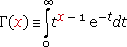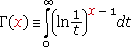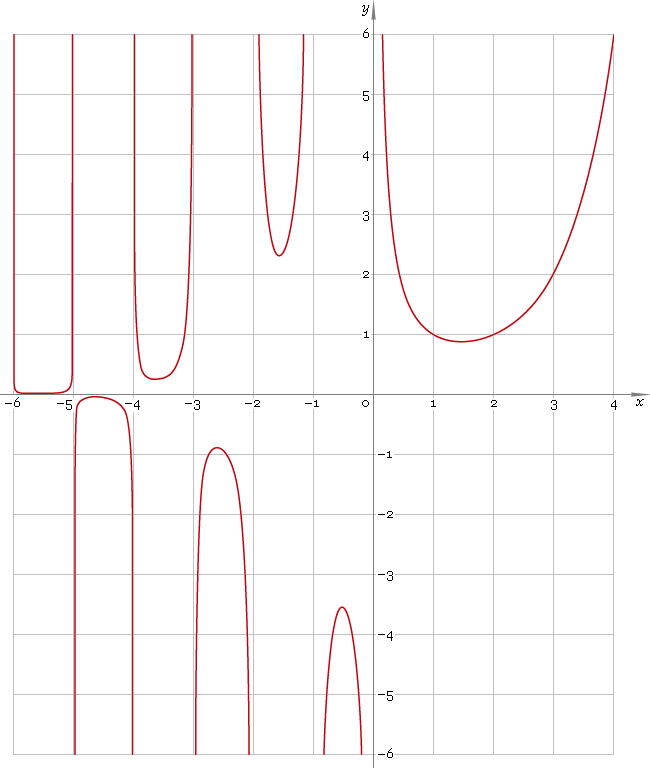The Art of Mathematics

# Γ or Gamma — gamma function

## 1. Definition

Gamma function is defined as integralor## 2. Graph

Gamma function defined everywhere on real axis, except its singular points n = 0, −1, −2, ... — so function domain is ...∪(−2, −1)∪(−1, 0)∪(0, +∞). Its graph is depicted below — fig. 1.Fig. 1. Graph of the gamma function y = Γ(x).

Function codomain is entire real axis except 0: (−∞, 0)∪(0, +∞).

## 3. Identities

Relation to factorial:

Γ(n) = (n − 1)!

Factorial-like properties:

Γ(x + 1) = x Γ(x) Γ(1 − x) = −x Γ(−x)

Extension to negative half-axis:

Γ(1 − x) = π/[Γ(x) sin(πx)]

Doulbe argument:

Γ(2x) = (2π)−1/2 22x − 1/2 Γ(x) Γ(x + 1/2)

Triple argument:

Γ(3x) = (2π)−1 33x − 1/2 Γ(x) Γ(x + 1/3) Γ(x + 2/3)

Γ(4x) = (2π)−3/2 44x − 1/2 Γ(x) Γ(x + 1/4) Γ(x + 1/2) Γ(x + 3/4)

Genaral formula for multiple argument:

Γ(nx) = (2π)(1−n)/2 nnx − 1/2 Γ(x) Γ(x + 1/n) ... Γ(x + (n − 1)/n)

Half-integer argument:

Γ(−5 /2) = −8 /15 √π
Γ(−3 /2) = 4 /3 √π
Γ(−1 /2) = −2 √π
Γ(1 /2) = √π
Γ(3 /2) = 1 /2 √π
Γ(5 /2) = 3 /4 √π

and in general:

Γ(1/2 + n) = (2n − 1)!! /2n√π = 1 × 3 × 5 × ... × (2n − 1) /2n√π

for negative values:

Γ(1/2 − n) = (−1)n2n/(2n − 1)!! √π = (−1)n2n/[1 × 3 × 5 × ... × (2n − 1)] √π

as well, for positive odd n:

Γ(n /2) = (n − 2)!! /2(n − 1)/2 √π

and for negative odd n:

Γ(n /2) = (−1)(n + 1)/2 2(n + 1)/2/n!! √π

## 4. Derivative

Gamma function derivative:

Γ′(z) = Γ(z) Ψ(z)

where Ψ is digamma function.

## 5. How to use

To calculate gamma function of the number:

``Γ(−1.5+i);``

or

``Gamma(−1.5+i);``

To get gamma function of the current result:

``Γ(rslt);``

or

``Gamma(rslt);``

To get gamma function of the number z in calculator memory:

``Γ(mem[z]);``

or

``Gamma(mem[z]);``

## 6. Support

Gamma function of the complex argument is supported in professional version of the Librow calculator.